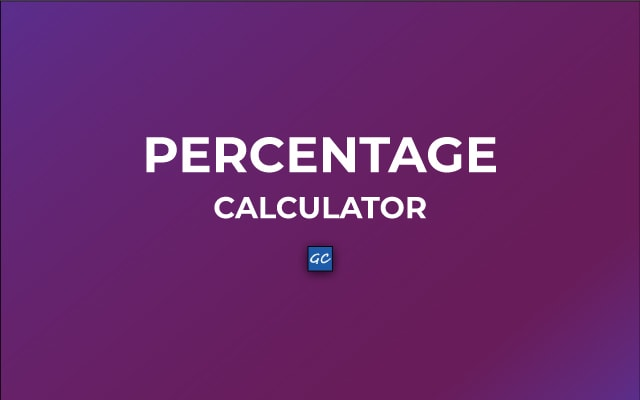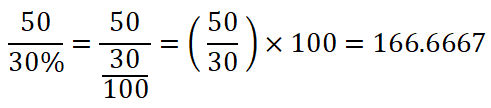# Percentage Calculator

Use this Percentage Calculator to find percent value online. Just need to enter two values inside the text boxes and press the “Calculate” button. As a result, you will get the final result very quickly.

 % × =
 What is % of ?
 is what percent of ? %
 is % of what?
 What is the percentage increase/decrease from to ? %
 Percent: Fraction: Decimal: %
RelatedMathematically, a Percentage can be defined as a number that is a fraction of 100. In short, a percentage is a part of 100.

In real life, we daily encounter percentages. Whether it’s the humidity percentage in the weather or your attendance percentage of classes. Also, The percentage is a score on the examination.

There are many applications of the percentage system. As it is one of the best ways to express a part of something. Here, we will showcase the best percentage calculator to make your percentage calculations easier and fast. Now no more hassles for manual calculation.

## Percentage Calculator

As mentioned above percentage is expressed as a fraction of 100.

For example, 30% is actually 30/100. Similarly, 45% represents 45/100.

This online tool has many functions. Thus, all together it becomes a one-stop destination for all types of percentage calculations.

Let’s take some examples to understand it. Also, there are many methods included for percent calculation. We will here discuss all of these.

#### Example1: What is the 40% of 1000?

These types of questions can easily be solved using this tool. There are input options to enter these values. Then in order to get the answer press “Calculate”. However, you should also know the mathematical process used behind it.

To know 40% of 1000. We will multiply 40% by 1000.

That is 40/100 x 1000 = 400.

This calculation looks simple but complex calculations will not be so easier. So, it will take much more time and effort. Therefore, it’s best to use the percentage calculating tool.

#### Example2: 60 is what percent of 180?

In this case, the mathematical explanation is:

Divide 60 by 180. After that multiply with 100%. As a result, you will get a percentage.

( 60/180 ) x 100% = 33.3333%

#### Example3: 50 is 30% of what?

So, divide 50 by 30% and the calculation is like this:Hence, 50 is 30% of 166.6667. Or you can also say 30% of 166.6667 is 50. Consequently, you can also compare the results in both ways.

#### Percentage Change

Not all Percentage Calculators have this feature. Undeniably, this is one of the best features of this tool. Surprisingly, it will take only a few seconds to do the calculation.

Generally, the expression used for determining the percentage change from X1 to X2 is:

Percentage Change = [(X2 – X1) / X1] x 100%

#### Example4: Let’s calculate the percentage change from 20% to 45%

Therefore, Percentage Change = (45-20)/20 x 100% = 125%.

Hence, there is a 125% change from 20% to 45%.

If sometimes X2 is less than X1. Then, in that case, the percentage change is negative.

#### Percentage to Fraction to Decimal using Percentage Calculator

Percentage Calculator allows converting the percentage into a fraction. Similarly, you can also convert that fraction into a decimal. This process goes in a reverse manner too. So, you can convert fractions to decimal or percentage and decimal to fraction or percent.

To do that just enter the value which you have. Then press the “=” (Equal to) button of the side which you want to convert. Like you wish to convert decimals to percentages. So, firstly enter the decimal value. Then press the “=” button which is between percentage and fraction.

### Conclusion

This Calculator is an online tool. Also, you need a device that can access the Internet. Then you can use it. Just open any Web Browser and go to the Percentage Calculator. After that decide the percentage calculation type you want to do. You can go above and see the steps again to use it.

This calculator gives many benefits as compared to manual calculations. When you go for manual calculation, time, and effort are wasted. Whereas on the other hand using this tool can save you valuable time and effort.

Furthermore, these calculators are very accurate. You can’t expect a computer to give a false output. These things together make this calculator the best among all online resources.